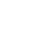# （电子书）Python基础知识：定义函数

``   def 函数名():``        代码``

``   # 定义一个函数，能够完成打印信息的功能``    def printInfo():``        print '------------------------------------'``        print 'Go语言进阶学习'``        print '------------------------------------'``

``  def add2num(a, b):``        c = a+b``        print c``

``def add2num(a, b):``    c = a + b``    print(c)``add2num(11, 22)# 调用带有参数的函数时，需要在小括号中，传递数据````>>> def test(a,b):``...     print(a,b)``... ``print(test(1, 2))``1 2``print(test(b=1, a=2))``2 1``>>> ``>>> print(test(b=1, 2))``  File "", line 1``SyntaxError: positional argument follows keyword argument````   # 定义完函数后，函数是不会自动执行的，需要调用它才可以``    printInfo()``

`` def add2num(a, b):``        c = a+b``        return c``

``   def add2num(a, b):``        return a+b``

``# 定义函数``def add2num(a, b):``    return a + b``# 调用函数，顺便保存函数的返回值``result = add2num(100, 98)``# 因为result已经保存了add2num的返回值，所以接下来就可以使用了``print(result)````print(my_abs('A'))````def my_abs(x):   ``  if not isinstance(x, (int, float)):     ``    raise TypeError('bad operand type')    ``    if x >= 0:      ``      return x  ``    else:      ``      return -x``

``my_abs('A')``《（电子书）Python基础知识：定义函数》